# Quantitative Aptitude Quiz for IBPS 2020 Mains Exams- 2nd December

Q1. In a bag, there are 16 balls of three different colors i.e. red, blue and green. Number of red and blue balls is 9 and difference between red and green ball is 4 then find the probability of getting a ball of each color if three balls are picked at random?
(a) 5/28
(b) 4/27
(c) 7/36
(d) 8/35
(e) 9/40

Q2. A bag contains only two colour balls i.e. red & green. Ratio of number of green balls to red balls is 4 : 3 is four green balls taken out from the bag, then the new ratio of green balls to red balls become 8 : 9. Find the probability of that if two balls taken out from the bags and both balls will be same colour?
(a) 17/35
(b) 17/38
(c) 14/35
(d) 12/35
(e) 11/35

Q3. An urn contains 4 red, 5 green, 6 blue and some yellow balls. If two balls are drawn at random, the probability of getting at least one yellow ball is 17/38. find the yellow balls in the urn.
(a) 4
(b) 5
(c) 6
(d) 10
(e) 15

Q4. An urn contains 6 red balls and 9 green balls. Two balls are drawn from the urn one
after other without replacement. Find the probability of drawing a red ball when a green
ball has been drawn from the urn.
(a) 3/15
(b) 3/7
(c) 9/14
(d) 7/11
(e) 2/5

Q5. Two dices are rolled simultaneously. Then, find probability that sum of numbers appearing on both dices is a multiple of 3.
(a) 1/9
(b) 1/12
(c) 1/4
(d) 1/3
(e) 1/6

Q6. A bag contains x green balls, 7 blue balls and 8 red balls. When two balls are drawn from bag randomly, then the probability of one ball being green and one ball being red is 4/15. Find value of x (number of green balls cannot be more than 18 balls).
(a) 3
(b) 5
(c) 10
(d) 9
(e) 15

Q7. In a bag, there are some red and total 9 black and yellow balls. Probability of selecting two red balls from that bag is 1/7 which is 250% of the probability of selecting two black balls. Find number of yellow balls in that bag if the number of black balls is even.
(a) 3
(b) 5
(c) 6
(d) 4
(e) None of these

Q8. Length of a rectangular land is twice the radius of a circle of circumference 132 cm. The land area got increased by 144 sq.cm, when a square land is attached along the breadth of the rectangle. Find area of rectangle in sq.cm.
(a) 1008
(b) 257
(c) 504
(d) 756
(e) 1512

Q9. Difference between circumference of a circular vessel (candy maker) and its diameter is 60 cm. If 40 cm² is waste material and 16 square boxes required to pack remaining quantity of candy, then find side of each square box?
(a) 4 cm
(b) 8 cm
(c) 10 cm
(d) 5 cm
(e) 6 cm

Q10. Perimeter of a rectangle is equal to the perimeter of a square whose side is twice of that of the breadth of the rectangle. What is the ratio of length of side of square to the length of rectangle?
(a) 4 : 9
(b) 6 : 5
(c) 7 : 9
(d) 14 : 9
(e) 2 : 3

Directions (11-12): The area of rectangular based tank, of which longer side is 150% more than smaller side is 1440 m² and the tank contains 10800 m³ water.

Q11. Total surface area of tank, if tank is opened from upper side.
(a) 2700 m²
(b)2400 m²
(c) 3600 m²
(d) 4900 m²
(e) 2100 m²

Q12. If radius of a conical tank is 7/8 th of smaller side of rectangular based tank and height is two times of height of rectangular based tank, then find capacity of water contained by conical tank?
(a) 6730 m³
(b) 6530 m³
(c) 6930 m³
(d) 6960 m³
(e) 6990 m³

Q13. A solid is in the form of a cylinder with hemispherical ends. If the height of the solid is 26cm and diameter of cylindrical part is 14 cm, then find the cost of covering the solid with metal sheet (Cost of metal sheet is Rs.15/cm²).
(a) Rs.16570
(b) Rs.15440
(c) Rs.17160
(d) Rs.12420
(e) Rs.14370

Q14. Find no. of same type of smaller spherical balls that can be formed from another hemisphere if radius of each such smaller spherical ball is 1/16 th of that hemisphere?
(a) 4096
(b) 2560
(c) 2048
(d) 5096
(e) 2296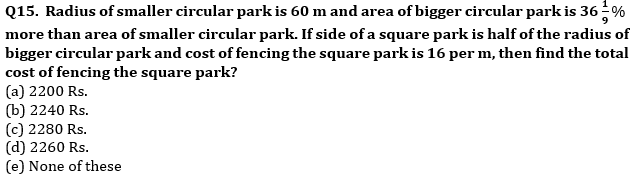Practice More Questions of Quantitative Aptitude for Competitive Exams:

Solutions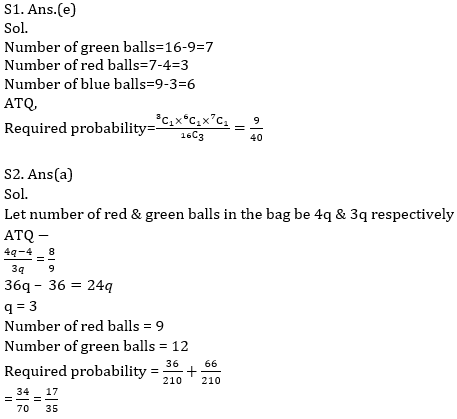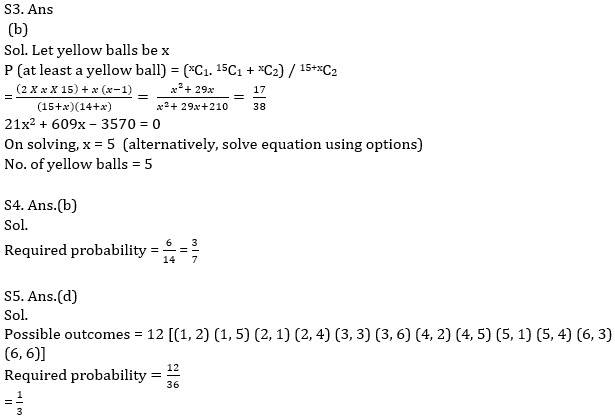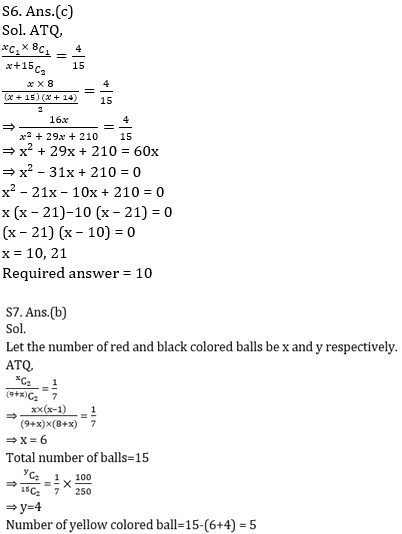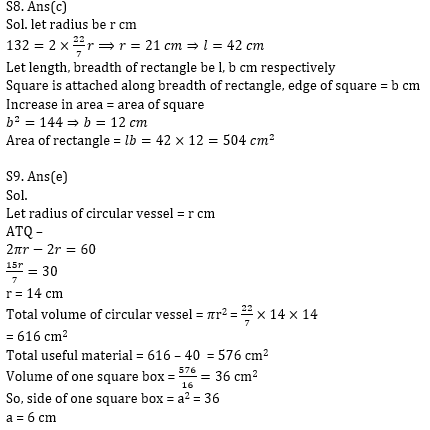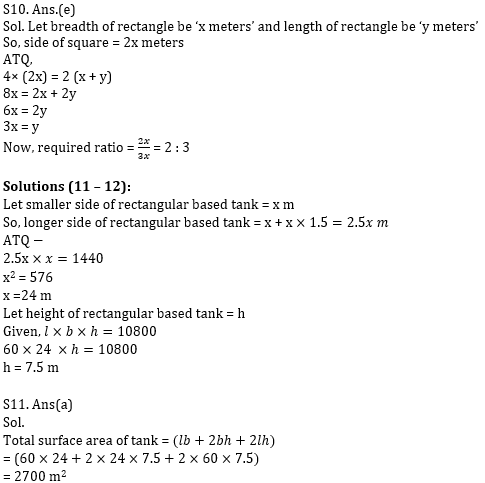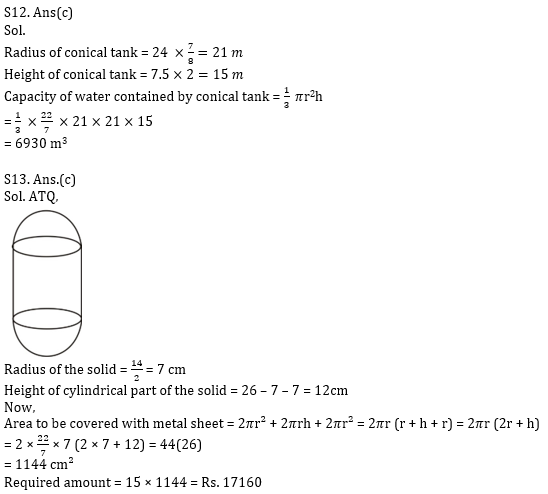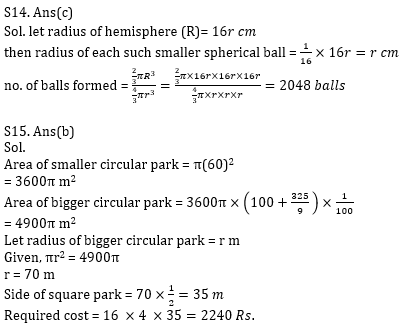Practice with Crash Course and Online Test Series for IBPS Clerk Prelims: